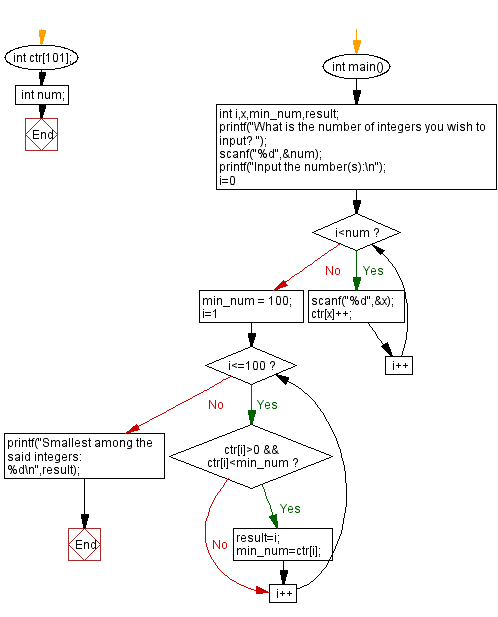﻿ C - Find the integer that appears the least often

# C Exercises: Find the integer that appears the least often

## C Basic-II: Exercise-1 with Solution

Write a C program that takes n number of positive integers. Find the integer that appears the least number of times among the said integers. If there are multiple such integers, select the smallest one.

Sample Date:

(1,2,3) -> 1
(10, 20, 4, 5, 11) -> 4

C Code:

``````#include <stdio.h>

int ctr;
int num;

int main()
{
int i,x,min_num,result;
printf("What is the number of integers you wish to input? ");
scanf("%d",&num);
printf("Input the number(s):\n");
for(i=0;i<num;i++)
{
scanf("%d",&x);
ctr[x]++;
}
min_num = 100;
for(i=1;i<=100;i++)
{
if( ctr[i]>0 && ctr[i]<min_num)
{  result=i;
min_num=ctr[i];
}
}
printf("Smallest among the said integers: %d\n",result);
}
``````

Sample Output:

```What is the number of integers you wish to input? 3
Input the number(s):
1
2
3
Smallest among the said integers: 1
```

Flowchart:C Programming Code Editor:

Previous C Programming Exercise: C Basic Part-II Exercises Home
Next C Programming Exercise: Reverse a string partially.

What is the difficulty level of this exercise?

Test your Programming skills with w3resource's quiz.

﻿